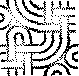#Combinatory Logic

Combinatory logic, or more correctly Iliative Combinatory Logic is a formal system closely related to LambdaCalculus.

The language of combinatory logic CL, consists of:

``` A finite set of variables taken from an infinite numerable set. x,y,z, ....

A combinator basis, S,K,I and the following reduction rules:

Ix=x
Kxy=x
Sfgx=fx(gx)
```
Combinatory Logic is easily translated to lambda calculus as follows:

• I is equivalent to the lambda calculus term (\x.x)

• K is equivalent to the lambda calculus term (\x.(\k.x))

• S is equivalent to the lambda calculus term (\f.(\g.(\x.fx(gx))))

The I combinator may be defined in terms of the SK combinators:

``` I=SKK=SKI
```
Proof by extension, i.e. applying SKK to x returns the same value as I applied to x.

SKKx reduces to Kx(Kx) which in turn reduces to x

SKSx reduces to Kx(Sx) which in turn reduces to x

There are several Combinatory Logics depending on the basis and reduction rules. There exists one combinator basis.

Although SK can generate the I combinator, there are some differences between the SK-Combinatory Logic and the SKI-Combinatory Logic.

A very good reference is the book about LambdaCalculus by HendrikBarendregt

Combinatory Logic allows to compile lazy functional programs very efficiently.

This was optimized by Turner in an article by 1979, where he presents an optimized basis to translate lambda calculus to combinatory logic.

Recursion is achieved by a fixed point combinator.

There are many fixed point combinators, all satisfy the equation

``` Fix f = f (Fix f)
```

This article should be extended to include the Turner Combinators, the Turner Abstractors. some examples of how to express some functions like the factorial, and more to relate to the lambda calculus, recursive functions. Combinatory Logic was intended to be a logic, but paradoxes related to Russell Paradox were discovered. It is a syntactic theory. HaskellCurry, Shoenfinkel, Feys, AlonzoChurch, are some of the main founders of this subject.EditText of this page (last edited April 26, 2010) or FindPage with title or text search